Command Reference : Operator and Function Listing

Operator and Function Listing
The following reference is an alphabetical listing of operators and functions which may be used in series assignment and generation, and in many cases, in matrix operations or element evaluation. Additional details on these operators and functions is provided in “Operator and Function Reference”

 Operator / Function Description + add – subtract * multiply / divide ^ raise to the power < less than <= less than or equal to <> not equal to = equal to > greater than >= greater than or equal to @abs(x), abs(x) absolute value @acos(x) arc cosine (real results in radians) @after(arg1) Creates a dummy variable equal to 1 if the observation is after or on the date given by arg1. arg1 should be enclosed in quotes. and logical and @asin(x) arc sine (real results in radians) @atan(x) arc tangent (results in radians) @before(arg1) Creates a dummy variable equal to 1 if the observation is before the date given by arg1. arg1 should be enclosed in quotes. @beta(a,b) beta integral @betainc(x,a,b[,n]) incomplete beta integral @betaincder(x,a,b,s) derivative of the incomplete beta integral @betaincinv(p,a,b) inverse of the incomplete beta integral @betalog(a,b) natural logarithm of the beta integral @between(x, val1, val2) Creates a dummy variable equal to 1 for observations where the series is greater than or equal to val1 and less than or equal to val2. @binom(n,x) binomial coefficient @binomlog(n,x) natural logarithm of the binomial coefficient @bounds(x,y,z) returns x if x is between y and z, and the boundary values y and z otherwise @bridge(x) returns a copy of series x with NAs replaced by the nearest preceding non-NA value. This function is panel aware. @cbeta(x,a,b[,n]) beta cumulative distribution (CDF) @cbvnorm(x, y, r) bivariate cumulative normal distribution (CDF) @cbinom(x,n,p) binomial cumulative distribution (CDF) @cchisq(x,v[,n]) chi-square cumulative distribution (CDF) @ceiling(x[,n]) smallest integer not less than @cellid element @cexp(x,m[,n]) exponential cumulative distribution (CDF) @cextreme(x[,n]) extreme value cumulative distribution (CDF) @cfdist(x,v1,v2[,n]) F-distribution cumulative distribution (CDF) @cgamma(x,b,r[,n]) gamma cumulative distribution (CDF) @cged(x,r[,n]) generalized error cumulative distribution (CDF) @chisq(x,v[,n]) chi-square p-value @claplace(x[,n]) Laplace cumulative distribution (CDF) @clogistic(x[,n]) logistic cumulative distribution (CDF) @cloglog(x) complementary log-log function @clognorm(x,m,s[,n]) log normal cumulative distribution (CDF) @cnegbin(x,n,p) negative binomial cumulative distribution (CDF) @cnorm(x[,n]) normal cumulative distribution (CDF) @columns(g) number of columns. @cor(x,y[,s]) correlation @cos(x) cosine (argument in radians) @cov(x,y[,s]) population covariance @covp(x,y[,s]) population covariance @covs(x,y[,s]) sample covariance @cpareto(x,a,k[,n]) Pareto cumulative distribution (CDF) @cpoisson(x,m) Poisson cumulative distribution (CDF) @crossid observations in range @ctdist(x,v) Student’s t-distribution cumulative distribution (CDF) @cumbmax(x[,s]) backwards cumulative maximum @cumbmean(x[,s]) backwards cumulative mean @cumbmin(x[,s]) backwards cumulative minimum @cumbnas(x[,s]) backwards cumulative nmber of NA observations @cumbobs(x[,s]) backwards cumulative nmber of non-NA observations @cumbprod(x[,s]) backwards cumulative product @cumbstdev(x[,s]) backwards cumulative sample standard deviation @cumbstdevp(x[,s]) backwards cumulative population standard deviation @cumbstdevs(x[,s]) backwards cumulative sample standard deviation @cumbsum(x[,s]) backwards cumulative sum @cumbsumsq(x[,s]) backwards cumulative sum-of-squares @cumbvar(x[,s]) backwards cumulative population variance @cumbvarp(x[,s]) backwards cumulative population variance @cumbvars(x[,s]) backwards cumulative sample variance @cumdn(x,d[,y,s]) cumulative negative (below threshold) changes @cumdp(x,d[,y,s]) cumulative positive (above threshold) changes @cumdz(x,d[,y,s]) cumulative zero (at threshold) changes @cummax(x[,s]) cumulative maximum @cummean(x[,s]) cumulative mean @cummin(x[,s]) cumulative minimum @cumnas(x[,s]) cumulative nmber of NA observations @cumobs(x[,s]) cumulative nmber of non-NA observations @cumprod(x[,s]) cumulative product @cumstdev(x[,s]) cumulative sample standard deviation @cumstdevp(x[,s]) cumulative population standard deviation @cumstdevs(x[,s]) cumulative sample standard deviation @cumsum(x[,s]) cumulative sum @cumsumsq(x[,s]) cumulative sum-of-squares @cumvar(x[,s]) cumulative population variance @cumvarp(x[,s]) cumulative population variance @cumvars(x[,s]) cumulative sample variance @cunif(x,a,b) uniform cumulative distribution (CDF) @cweib(x,m,a[,n]) Weibull cumulative distribution (CDF) d(x) first difference d(x,n) n-th order difference d(x,n,s) n-th order difference with a seasonal difference at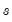@date element @dateadd(d, offset[,u]) calculates date number offsets @dateceil(d1, u[, step] calculates the last possible date number for a date with a given time unit @datediff(d1,d2[,u]) difference between two dates @datefloor(d1, u[, step]) calculates a date floor @dateceil(d1, u[, step] calculates the next date number for a date with a given time unit @datepart(d1, u) calculates the date part of a number @datestr(d[, fmt]) converts date to string @dateval(str1[, fmt]) converts string to date @day day of month @dbeta(x,a,b) beta density function @dbinom(x,n,p) binomial density function @dbname returns a string containing the current default database name @dbvnorm(x,y,r) bivariate normal density function @dchisq(x,v) chi-square density function @dcumdn(x,d[,y,s]) addition to cumulative negative (below threshold) changes @dcumdp(x,d[,y,s]) addition to cumulative positive (above threshold) changes @dcumdz(x,d[,y,s]) addition cumulative zero (at threshold) changes @demean(x[, s]) returns a copy of series x translated to have a mean of zero @demeanby(x, g[, s]) returns a copy of series x translated to have a mean of zero within each group of observations defined by series g. @detrend returns the residuals of an OLS regression on series x versus an implicit time trend. This function is panel aware. @dexp(x,m) exponential density function @dextreme(x) extreme value density function @dfdist(x,v1,v2) F-distribution density function @dgamma(x,b,r) gamma density function @dged(x,r) generalized error density function @digamma(x), @psi(x) first derivative of the log gamma function @diwish([v,]s) Inverse Wishart density with sym covariance @diwishc([v,]s) Inverse Wishart density with sym Choesky of covariance @diwishi([v,]s) Inverse Wishart density with sym inverse covariance @diwishic([v,]s) Inverse Wishart density with sym Cholesky of inverse covariance @dlaplace(xb) Laplace density function dlog(x) first difference of the logarithm dlog(x,n) n-th order difference of the logarithm dlog(x,n,s) n-th order difference of the logarithm with a seasonal difference at@dlogistic(x) logistic density function @dlognorm(x,m,s) log normal density function @dmvnorm([v,]s) multivariate normal density with sym covariance @dmvnormc([v,]s) multivariate normal density with sym Choesky of covariance @dmvnormi([v,]s)i multivariate normal density with sym inverse covariance @dmvnormic([v,]s) multivariate normal density with sym Cholesky of inverse covariance @dnegbin(x,n,p) negative binomial density function @dnorm(x) normal density function @dpareto(x,a,k) Pareto density function @dpoisson(x,m) Poisson density function @dtdist(x,v) Student’s t-distribution density function @dtoo(str) converts string to observation number @dunif(x,a,b) uniform density function @dupselem(x1[, x2,..., s]) duplicates enumeration @dupsid(x1[, x2,..., s]) duplicates identifiers @dupsobs(x1[, x2,..., s]) duplicates observations @during(arg1) Creates a dummy variable equal to 1 if the observation lies between the dates given by the date pair contained in arg1, and 0 otherwise. arg1 should be given in quotes. @dweib(x,m,a) Weibull density function @dwish([v,]s) Wishart density with sym covariance @dwishc([v,]s) Wishart density with sym Choesky of covariance @dwishi([v,]s) Wishart density with sym inverse covariance @dwishic([v,]s) Wishart density with sym Cholesky of inverse covariance @elem(x,"v") element @enddate observations in range @env(str) returns a string containing the value of the Windows environment variable str. @eqna(str1, str2) test for equality of strings @eqna(x,y) equal to @erf(x) error function @erfc(x) complementary error function @event(arg1[, basis]) Proportion of a one-off event that lies in each observation. @exp(x), exp(x) exponential,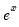@expm1(x) for x near zero,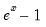@fact(x) factorial,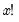@factlog(x) natural logarithm of the factorial,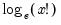@fdist(x,v1,v2) F-distribution p-value @fileexist(str) returns a 0 or 1 depending on whether the filename specified by str exists on disk. @first(x) returns the value of the first non-missing value in the series for the current sample. @firstsby(arg1, arg2[, s]) First non-missing value in arg1 for each arg2 group. @floor(x[,n]) largest integer not greater than. @folderexist(str) returns a binary scalar, equal to 1 if the folder specified by str exists on disk, and 0 otherwise. @fracdiff fractional difference operator. @fv(r,n,x[,fv,t]) future value. @gamma(x) (complete) gamma function @gammader(x) first derivative of the gamma function @gammainc(x,a[,n]) incomplete gamma function @gammaincder(x,a,n) derivative of the incomplete gamma function @gammaincinv(p,a) inverse of the incomplete gamma function @gammalog(x) logarithm of the gamma function @getnextname(str) returns a string containing the next available variable name in the workfile, starting with str. e.g. entering “result” will return “result01” unless there is already a RESULT01, in which case it will return “result02”. @gmean(x[,s]) geometric mean @groupid(arg1[, arg2, ..., argn, s]) group index for the groups defined by arg1 to argn. @hmean(x[.s]) harmonic mean @holiday(h[, b][, flag...]) Proportion of an annual event that lies in each observation. @holidayset(h[, b][, flag...]) Proportion of annual events that lies in each observation. @hour returns the hour of each observation as an integer. @hourf returns the hour of each observation as a floating point number. @iff(s,x,y) recode by condition @ifirst(x) returns the workfile index of the first non-missing value in the series for the current sample. @ilast(x) returns the workfile index of the last non-missing value in the series for the current sample. @imax(x) returns the workfile index of the maximum value in the series for the current sample. @imin(x) returns the workfile index of the minimum value in the series for the current sample. @inlist(x, “list”) Creates a dummy variable equal to 1 for observations where the series is equal to one of the values specified in list, and 0 otherwise. list should be a quoted, space delimited list of values. This function works on both numerical and alpha series. @inner(x,y[,s]) inner product @insert(str1, str2, n]) string insertion @instr(str1, str2[, n]) string location @intercept(x[,s]) intercept of OLS regression versus implicit time trend @inv(x) reciprocal,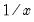@isempty(str) tests for blank strings @isna(x) equal to NA @ispanel returns indicator for whether the workfile is panel structured. @isperiod(x) period dummy @kurt(x[,s]) kurtosis @kurtsby(arg1, arg2[,arg3, arg4..., argn, s]) kurtosis of arg1 observations for each arg2 to argn defined group. @lag(x[, n]) n-th order lag (equivalent to “X(‑4”) for the series X) @last(x) returns the value of the last non-missing value in the series for the current sample. @lastsby(arg1, arg2[, s]) Last non-missing value in arg1 for each arg2 group. @left(str, n) returns the left part of a string @len(str) length of a string @length(str) length of a string @log(x), log(x) natural logarithm,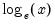@log10(x) base-10 logarithm,@log1mexp(x) for negative x near zero,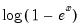@log1p(x) for x near zero,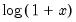@log2pi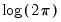@logcnorm @log(@cnorm(x)) @logit(x) logistic transform @logx(x,b) base-b logarithm,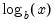@lower(str) lowercases a string @ltrim(str) removes spaces from a string @mae(x, y) the mean of the absolute value of the difference between X and Y. @makedate(arg1[,arg2[,arg3]], fmt]) converts number to date @map(x[, mapname]) mapped value @mape(x,y) 100 multiplied by the mean of the absolute difference between X and Y, divided by Y. @mav(x,n) n-period backward moving average. NAs are not propagated. @mavc(x,n) n-period centered moving average. NAs are not propagated. @max(x[,s]) maximum @maxes(x,n) n-maximum values, returned largest to smallest in a vector object. @maxsby((arg1, arg2[, arg3, arg4..., argn, s) maximum value of arg1 observations for each arg2 to argn group. @mcor(x,y,n) n-period backwards moving correlation. NAs are not propagated. @mcov(x,y,n) n-period backwards population moving covariance. NAs are not propagated. @mcovp(x,y,n) n-period backwards moving population covariance. NAs are not propagated. @mcovs(x,y,n) n-period backwards moving sample covariance. NAs are not propagated. @mean(x[,s]) mean @meansby(arg1, arg2[,arg3, arg4..., argn, s]) mean of arg1 observations for each arg2 to argn defined group. @median(x[,s]) median @mediansby(arg1, arg2[, arg3, arg4..., argn, s]) median of arg1 observations for each arg2 to argn defined group @mid(str, n1[, n2]) returns the middle part of a string @min(x[,s]) minimum @minner(x,y,n) n-period backwards inner product of X and Y. NAs are not propagated. @mins(x,n) n-minimum values, returned smallest to largest in a vector object. @minsby(arg1, arg2[,arg3, arg4..., argn, s]) minimum value of arg1 observations for each arg2 to argn defined group. @minute returns the minute of each observation as an integer. @mkurt(x,n) n-period backwards kurtosis. NAs are not propagated. @mmax(x,n) n-period backwards moving maximum. NAs are not propagated. @mmedian(x,n) n-period backward moving median. NAs are not propagated. @mmin(x,n) n-period backwards moving minimum. NAs are not propagated. @mnas(x,n) n-period backwards number of NA observations. NAs are not propagated. @mobs(x,n) n-period backwards number of non-NA observations. NAs are not propagated. @mod(x,y) floating point remainder @month month @movav(x,n) n-period backward moving average. NAs are propagated. @movavc(x,n) n-period centered moving average. NAs are propagated. @movcor(x,y,n) n-period backwards moving correlation. NAs are propagated. @movcov(x,y,n) n-period backwards moving population covariance. NAs are propagated. @movcovp(x,y,n) n-period backwards moving population covariance. NAs are propagated. @movcovs(x,y,n) n-period backwards moving sample covariance. NAs are propagated. @movinner(x,y,n) n-period backwards inner product of X and Y. NAs are propagated. @movkurt(x,n) n-period backwards kurtosis. NAs are propagated. @movmax(x,n) n-period backwards moving maximum. NAs are propagated. @movmin(x,n) n-period backwards moving minimum. NAs are propagated. @movnas(x,n) n-period backwards nmber of NA observations @movobs(x,n) n-period backwards nmber of non-NA observations @movskew(x,n) n-period backwards skewness. NAs are propagated. @movstdev(x,n) n-period backwards moving sample standard deviation. NAs are propagated. @movstdevp(x,n) n-period backwards moving population standard deviation. NAs are propagated. @movstdevs(x,n) n-period backwards moving sample standard deviation. NAs are propagated. @movsum(x,n) n-period backward moving sum. NAs are propagated. @movsumsq(x,n) n-period backwards sum-of-squares. NAs are propagated. @movvar(x,n) n-period backwards moving population variance. NAs are propagated. @movvarp(x,n) n-period backwards moving population variance. NAs are propagated. @movvars(x,n) n-period backwards moving sample variance. NAs are propagated. @mse(x,y) the mean of the squared difference between X and Y. @mskew(x,n) n-period backwards skewness. NAs are not propagated. @mstdev(x,n) n-period backwards moving sample standard deviation. NAs are not propagated. @mstdevp(x,n) n-period backwards moving population standard deviation. NAs are not propagated. @mstdevs(x,n) n-period backwards moving sample standard deviation. NAs are not propagated. @msum(x,n) n-period backward moving sum. NAs are not propagated. @msumsq(x,n) n-period backwards sum-of-squares. NAs are not propagated. @mvar(x,n) n-period backwards moving population variance. NAs are not propagated. @mvarp(x,n) n-period backwards moving population variance. NAs are not propagated. @mvars(x,n) n-period backwards moving sample variance. NAs are not propagated. @nan(x,y) recode NAs in X to Y @nas(x[,s]) number of NAs @nasby(arg1, arg2[,arg3, arg4..., argn, s]) number of arg1 NA values for each arg2 to argn defined group. @neqna(str1, str2) tests for inequality of strings @neqna(x,y) not equal to @now returns current time @nper(r,x,pv[,fv,t]) annuity number of periods nrnd normal random number generator @obs(x[,s]) number of observations @obsby(arg1, arg2[,arg3, arg4..., argn, s]) number of non-NA arg1 observations for each arg2 to argn defined group. @obsid cross-section observation number @obsrange observations in range @obssmpl observations in sample or logical or @otod(n) converts observation number to string @otods(n) converts sample observation number to string @pagecount returns a scalar containing the number of pages in the current workfile. @pageexist(str) returns a 0 or 1 depending on whether the page specified by str exists in the current workfile. @pagefreq returns a string containing the current page’s frequency. @pageids returns a string containing a space delimited list of the id series for the current workfile page. @pagelist returns a space delimited string containing the names of all the pages in the current active workfile. @pagename returns a string containing the current default page name. @pagesmpl returns a string containing the current sample for the active page. @pc(x) one-period percentage change (in percent) @pca(x) one-period percentage change—annualized (in percent) @pch(x) one-period percentage change (in decimal) @pcha(x) one-period percentage change—annualized (in decimal) @pchy(x) one-year percentage change (in decimal) @pctiles(x[, ties, s]) percentile @pcy(x) one-year percentage change (in percent) @periodtodate(x, p) cumulative sum of series x within each period, where each period is defined by a contiguous block of identical values in series p @pi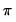@pmax(x,y) returns the pairwise max of x and y (for more than pairs, you may still use @rmax) @pmin(x,y) returns the pairwise min of x and y (for more than pairs, you may still use @rmin @pmt(r,n,pv[,fv,t]) payment amount @pow(x,a) power,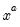@powm1(x,a) for x near 1,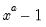@pow1pm1(x,a) for x near zero,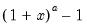@prod(x[,s]) product @psi(x) see @digamma @pv(r,n,x[,fv,t]) present value @qbeta(s,a,b) beta quantile function (inverse CDF) @qbinom(s,n,p) binomial quantile function (inverse CDF) @qchisq(p,v) chi-square quantile function (inverse CDF) @qexp(p,m) exponential quantile function (inverse CDF) @qextreme(p) extreme value quantile function (inverse CDF) @qfdist(p,v1,v2) F-distribution quantile function (inverse CDF) @qgamma(p,b,r) gamma quantile function (inverse CDF) @qged(p,r) generalized error quantile function (inverse CDF) @qlaplace(x) Laplace quantile function (inverse CDF) @qlogistic(p) logistic quantile function (inverse CDF) @qlognorm(p,m,s) log normal quantile function (inverse CDF) @qnegbin(s,n,p) negative binomial quantile function (inverse CDF) @qnorm(p) normal quantile function (inverse CDF) @qpareto(p,a,k) Pareto quantile function (inverse CDF) @qpoisson(p,m) Poisson quantile function (inverse CDF) @qtdist(p,v) Student’s t-distribution quantile function (inverse CDF) @quantile(x,q[,m,s]) quantile @quantilesby(arg1, arg2[,arg3, arg4..., argn], q[, s]) q-quantiles of arg1 observations for each arg2 to argn defined group. @quarter quarter of the year in a dated workfile @qunif(p,a,b) uniform quantile function (inverse CDF) @qweib(p,m,a) Weibull quantile function (inverse CDF) @ranks(x[,o,t,s]) rank @rate(n,x,pv[,fv,t]) interest @rbeta(a,b) beta random number generator @rbinom(a,b) binomial random number generator @rchisq(v) chi-square random number generator @recode(s,x,y) recode by condition @replace(str1, str2, str3[,n]) replaces a string with another @rexp(m) exponential random number generator @rextreme(p) extreme value random number generator @rfdist(v1,v2) F-distribution random number generator @rfirst(g) row-wise first non-NA value. @rgamma(b,r) gamma random number generator @rged(r) generalised error random number generator @right(str,n) returns the right parts of a string @rifirst(g) row-wise first non-NA index. @rilast(g) row-wise last non-NA index. @rimax(g) row-wise maximum index. @rimin(g) row-wise minimum index. @riwish([v,]s) Inverse Wishart random number generator with sym covariance @riwishc([v,]s) Inverse Wishart random number generator with sym Choesky of covariance @riwishi([v,]s)i Inverse Wishart random number generator with sym inverse covariance @riwishic([v,]s) Inverse Wishart andom number generator with sym Cholesky of inverse covariance @rlaplace Laplace random number generator @rlast(g) row-wise last non-NA value. @rlogistic logistic random number generator @rlognorm(m,s) log normal random number generator @rmax(g) row-wise maximum value. @rmean(g) row-wise mean. @rmedian(g) row-wise median @rmin(g) row-wise minimum value. @rmvnorm([v,]s) multivariate normal random number generator with sym covariance @rmvnormc([v,]s) multivariate normal random number generator with sym Choesky of covariance @rmvnormi([v,]s)i multivariate normal random number generator with sym inverse covariance @rmvnormic([v,]s) multivariate normal random number generator with sym Cholesky of inverse covariance @rnas(g) row-wise number of NAs. @rnegbin(n,p) negative binomial random number generator @rnorm normal random number generator @robs(g) row-wise number of non-NAs. @round(x[,n]) round to the nearest integer @rpareto(a,k) Pareto random number generator @rpoisson(m) Poisson random number generator @rprod(g) row-wise product (one should be careful of overflow) @rquantile(g,q) row-wise quantile, where q is the quantile. The Cleveland quantile definition is used. @rstdev(g) row-wise sample standard deviation. @rstdevp(g) row-wise population standard deviation. @rstdevs(g) row-wise sample standard deviation. @rsum(g) row-wise sum. @rsumsq(g) row-wise sum-of-squares. @rtdist(v) Student’s t-distribution random number generator @rtrim(str) removes spaces from a string @runif(a,b) uniform random number generator @rvalcount(g,v) row-wise count of observations matching v. @rvar(g) row-wise population variance. @rvarp(g) row-wise population variance @rvars(g) row-wise sample variance. @rweib(m,a) Weibull random number generator @rwish([v,]s) Wishart random number generator with sym covariance @rwishc([v,]s) Wishart random number generator with sym Choesky of covariance @rwishi([v,]s)i Wishart random number generator with sym inverse covariance @rwishic([v,]s) Wishart andom number generator with sym Cholesky of inverse covariance @seas(x) seasonal dummy @second returns the second of each observation as an integer. @sign(x) returns -1, 0, 1 depending on the sign of the corresponding element of x @sin(x) sine (argument in radians) @skew(x[,s]) skewness @skewsby(arg1, arg2[,arg3, arg4..., argn, s) skewness of arg1 observations for each arg2 to argn defined group. @smape(x,y[,s]) symmetric mean absolute percentage error @sqrt(x), sqr(x) square root @stdev(x[,s]) standard deviation @stdevp(x[,s]) population standard deviation @stdevs(x[,s]) sample standard deviation @stdevpsby(arg1, arg2[,arg3, arg4..., argn, s]) standard deviation of arg1 observations for each arg2 to argn defined group (division by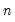). @stdevsby(arg1, arg2[,arg3, arg4..., argn, s]) sample standard deviation of arg1 observations for each arg2 to argn defined group (division by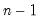). @stdevssby((arg1, arg2[,arg3, arg4..., argn, s]) sample standard deviation of arg1 observations for each arg2 to argn defined group (division by). @stdize(x[, s]) standardize series using sample variance. @stdizep(x[,s]) standardize series using population variance. @str(d,[fmt]) converts a number to a string @strdate(fmt) string corresponding to each element in workfile @strdate(x) string dates @strlen(str) length of a string @strnow(fmt) returns current time as a string @sum(x[,s]) sum @sumsby(arg1, arg2[, s]) sum of arg1 observations for each arg2 to argn defined group. @sumsq(x[,s]) sum-of-squares @sumsqsby(arg1, arg2[, s]) sum of squares of arg1 observations for each arg2 to argn defined group. @tablenames(str) returns a space delimited string containing the names of the table names of a foreign file. @tan(x) tangent (argument in radians) @tdist(x,v) t-distribution p-value @trend time trend @trend([x]) time trend @trendc calendar time trend @trendc([x]) calendar time trend @trendcoef(x[,s]) coefficient from OLS regression on a trend @trmean(x,p[, s]) trimmed mean @trigamma(x) second derivative of the log gamma function @trim(str) removes spaces from a string @unmap(x, mapname) unmapped numeric value @unmaptxt(x, mapname) unmapped text value @upper(str) uppercases a string @val(str[, fmt]) converts a string to a number @var(x[,s]) population variance @varp(x[,s]) population variance @varpsby(arg1, arg2[,arg3, arg4..., argn, s]) variance of arg1 observations for each arg2 to argn defined group (division by). @vars(x[,s]) sample variance @varsby(arg1, arg2[,arg3, arg4..., argn, s]) variance of arg1 observations for each arg2 to argn defined group (division by). @varssby(arg1, arg2[,arg3, arg4..., argn, s]) sample variance of arg1 observations for each arg2 to argn defined group (division by). @vernum returns a scalar containing the EViews version number. @verstr returns a string containing the EViews version string (EViews Enterprise Edition). @wdir(str) returns a string list containing a list of all of the files in the directory str. @weekday day of the week @wfname returns a string containing the current default workfile name. @wfpath returns a string containing the current default workfile path. @wquery("database", "search_expression", "attribute_list") returns a string list containing the attribute_list of all objects in database that match the query given by search_expression . @year year @xgetstr(string) returns a string from the external application. @xgetnum(string) returns a scalar from the external application. @xverstr returns the external application version number as a string. @xvernum returns the external application version number as a number. @ytd(x, p) returns the cumulative sum of a series within the year.